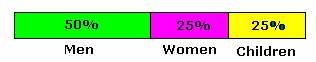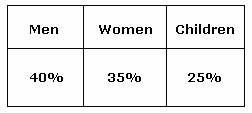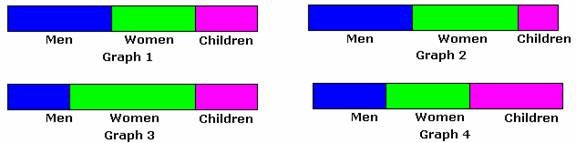## Definition Of Divided Bar Graph

In a Divided Bar Graph, a bar is divided into several segments to represent a set of quantities according to the different proportions of the total amount.

### More About Divided Bar Graph

The concept of divided bar graph is similar to that of a pie chart.
A divided bar graph can be either horizontal or vertical.

### Example of Divided Bar Graph

The following divided bar graph shows the percentage of men, women, and children who visited a zoological park out of the total people.Green color indicates the percentage of men who visited the park; pink color indicates the percentage of women; and yellow color indicates the percentage of children.

### Solved Example on Divided Bar Graph

#### Ques: The population of a city (in percent) is shown in the table.#### Which of the divided bar graphs represents the given table?A. Graph 1
B. Graph 2
C. Graph 3
D. Graph 4